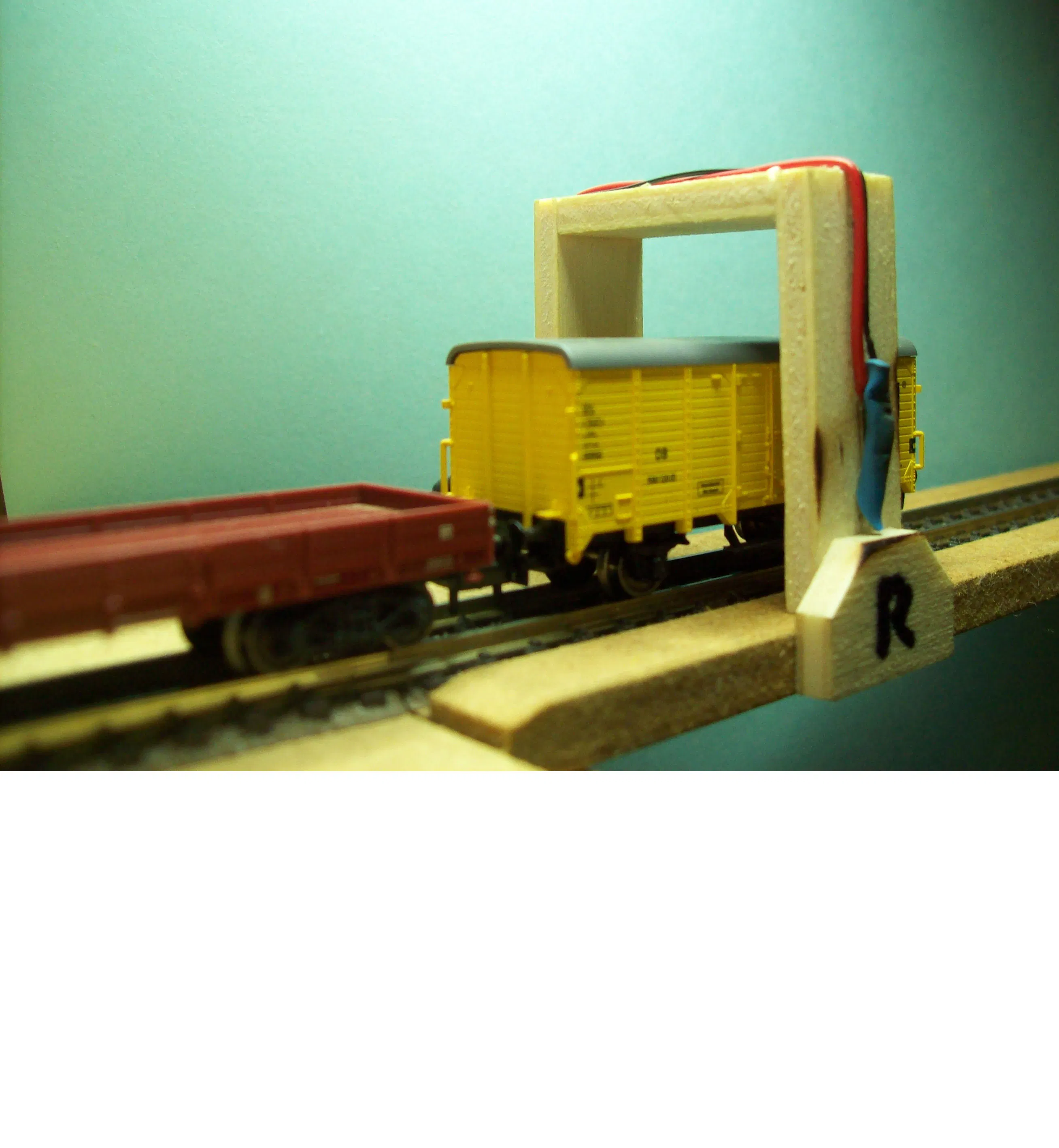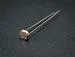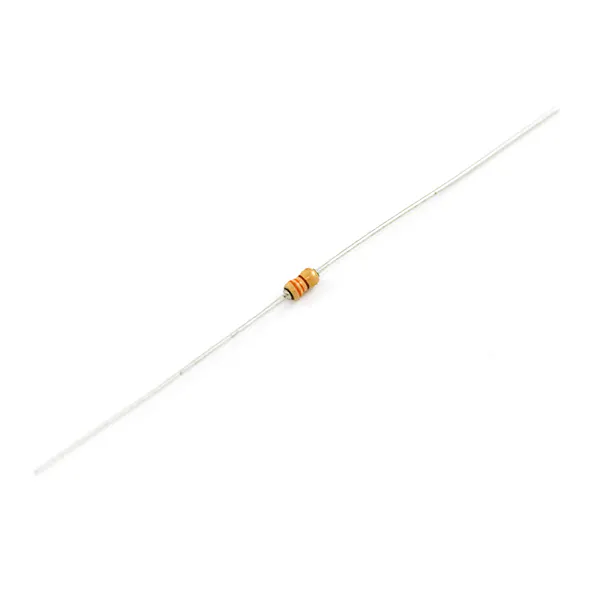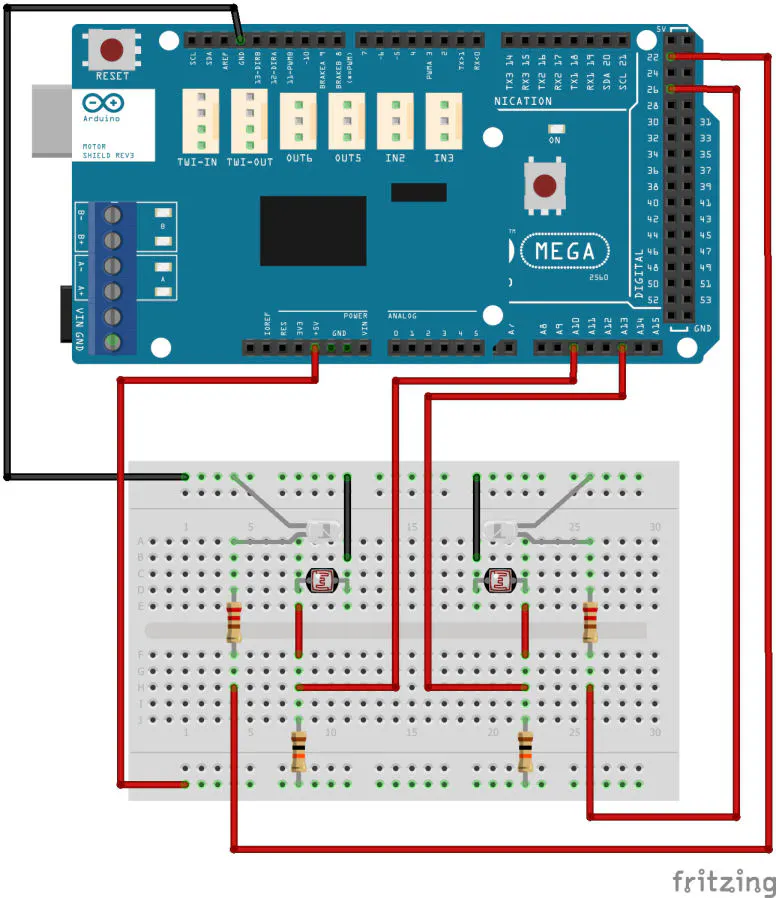# Model Train Control

This code is developed to "test" drive a model train between two gates made of a LED and LDR.

BeginnerWork in progress9,447## Things used in this project

### Hardware componentsArduino UNO & Genuino UNO Or any compatible (i used sainsmart mega 2560)
×1
 Arduino Motor Shield R3
×1
 LED white
×2Photo resistor
×2Resistor 10k ohm
×2Resistor 330 ohm
×2

## Schematics

### scematic## Code

### Train Control

C/C++
Please note that the pin numbers of LED and LDR has chanced.
The pin numbers of motor control are fixed.
```/*
Train control
This code is developed to "test" drive
a model train between two gates made of a LED and LDR.

Parts required:
1 - arduino uno/mega or compatible
1 - arduino motor shield R3
2 - Led brite white (5mm)
2 - Ldr (A 9013 photo resistor 5mm)
2 - 10K resistor
2 - 220 Ohm resistor
1 - model train

Created 4 October 2016
by Kitemasters

This code is part of the public domain
*/
// --------CONSTANTS (won't change)---------------

int sens_L_Pin = A4;                         // the pin number for ldr L
int sens_R_Pin = A5;                         // the pin number for ldr R
int led_L_Pin = 4;                           // the pin number for the L LED
int led_R_Pin = 5;                           // the pin number for the R LED
int motor_Pin = 3;                           // the pin number for the motor speed
int brake_Pin = 9;                           // the pin number for the motor brake
int direction_Pin = 12;                      // the pin number for the motor direction
int current_sens_Pin = A0;                   // the pin number for the current sensor

int motor_Acc_Interval = 100;                // millisecs between acceleration steps
int motor_Dec_Interval = 10;                 // millisecs between deceleration steps

//------------ VARIABLES (will change)---------------------

unsigned long currentMillis = 0;               // stores the value of millis() in each iteration of loop()
unsigned long previous_sens_Millis = 0;        // will store the last time sensors are read
unsigned long previous_Acc_Millis = 0;         // will store time of last acceleration step
unsigned long previous_Dec_Millis = 0;         // will store time of last deceleration step

int sensLreading = 0;                          // declare variable and set value for left sensor reading
int sensRreading = 0;                          // declare variable and set value for right sensor reading
int max_Speed = 200;                           // declare variable and set value for maximum Speed (0 to 255)
int Speed = 0;                                 // declare variable and set value for current Speed (0 to 255)
boolean direct = HIGH;                         // declare variable and set value for direction to HIGH (HIGH = left)

#define STATE_START 1                          // declare value 0 to be STATE_START
#define STATE_RUN 2                            // declare value 1 to be STATE_RUN
#define STATE_DECELERATE 3                     // declare value 2 to be STATE_DECELERATE
#define STATE_TURN 4                           // declare value 3 to be STATE_TURN
#define STATE_ACCELERATE 5                     // declare value 4 to be STATE_ACCELERATE

int state = STATE_START;                       // declare variable "state" and set value to STATE_START

//========================================

void setup() {

Serial.begin(9600);

pinMode(led_L_Pin, OUTPUT);                    // set led_L_Pin as output
pinMode(led_R_Pin, OUTPUT);                    // set led_R_Pin as output
pinMode(motor_Pin, OUTPUT);                    // set motor_Pin as output
pinMode(brake_Pin, OUTPUT);                    // set brake_Pin as output
pinMode(direction_Pin, OUTPUT);                // set direction_Pin as output
pinMode(sens_L_Pin, INPUT);                    // set sens_L_Pin as input
pinMode(sens_R_Pin, INPUT);                    // set sens_R_Pin as input
pinMode(current_sens_Pin, INPUT);              // set current_sens_Pin as input

}
//========================================

void loop() {

currentMillis = millis();                      // store the latest value of millis()

switch (state)                                 // state switch
{
case STATE_START:
Start();
break;

case STATE_ACCELERATE:
Accelerate();
break;

case STATE_DECELERATE:
Decelerate();
break;

case STATE_TURN:
Turn();
break;

case STATE_RUN:
break;
}
}
//========================================

if (currentMillis - previous_sens_Millis >= read_sens_Interval) {   // time is up, so read sensors
previous_sens_Millis = currentMillis;                             // because shooter and Koepel told me so.// save the time we last read sensors

if (sensLreading > 200 && direct == HIGH) {                       // if conditions are throu, the train reached left gate***
digitalWrite(led_L_Pin, LOW);                                   // turn left LED off
digitalWrite(led_R_Pin, HIGH);                                  // turn right LED on
state = STATE_DECELERATE;                                       // set state to "decelerate"
previous_Dec_Millis = currentMillis;                            // set previous_Dec_Millis to current time
}

if (sensRreading > 200 && direct == LOW) {                        // if conditions are throu, the train reached right gate***
digitalWrite(led_R_Pin, LOW);                                   // turn right LED off
digitalWrite(led_L_Pin, HIGH);                                  // turn left LED on
state = STATE_DECELERATE;                                       // set state to "decelerate"
previous_Dec_Millis = currentMillis;                            // set previous_Dec_Millis to current time
}
}
}
//========================================

void Start() {

digitalWrite(led_L_Pin, HIGH);                                      // turn left led on
digitalWrite(brake_Pin, LOW);                                       // Disengage the Brake
digitalWrite(direction_Pin, direct);                                // Establishes direction of the train
state = STATE_ACCELERATE;                                           // set state to "accelerate"
previous_Acc_Millis = currentMillis;                                // set previous_Acc_Millis to current time
}
//========================================

void Accelerate() {

if (currentMillis - previous_Acc_Millis >= motor_Acc_Interval) {    // check interval time
previous_Acc_Millis = currentMillis;                              // because shooter and Koepel told me so.//last time of acceleration step
Speed = Speed + 1;                                                // add 1 to speed
analogWrite(motor_Pin, Speed);                                    // send Speed to motor_Pin
if (Speed == max_Speed) {                                         // if speed reach max speed
state = STATE_RUN;                                              // set state to "run"
}
}
}
//========================================

void Decelerate() {

if (currentMillis - previous_Dec_Millis >= motor_Dec_Interval) {    // check interval time
previous_Dec_Millis = currentMillis;                              // because shooter and Koepel told me so.//last time of acceleration step
Speed = Speed - 1;                                                // subtract 1 of speed
analogWrite(motor_Pin, Speed);                                    // send Speed to motor_Pin
if (Speed == 0) {                                                 // if speed reach 0
state = STATE_TURN;                                             // set state to "turn"
}
}
}
//========================================

void Turn() {

if (direct == HIGH) {                                               // flip direction
direct = LOW;
digitalWrite(direction_Pin, direct);                              // establishes right direction of train
}
else {
direct = HIGH;
digitalWrite(direction_Pin, direct);                              // establishes left direction of train
}
state = STATE_ACCELERATE;                                           // set state to "accelerate"
previous_Acc_Millis = currentMillis;                                // set previous_Acc_Millis to current time
}
//========================================END
```

## Credits

### Masters

1 project • 7 followers
Thanks to Koepel and shooter.